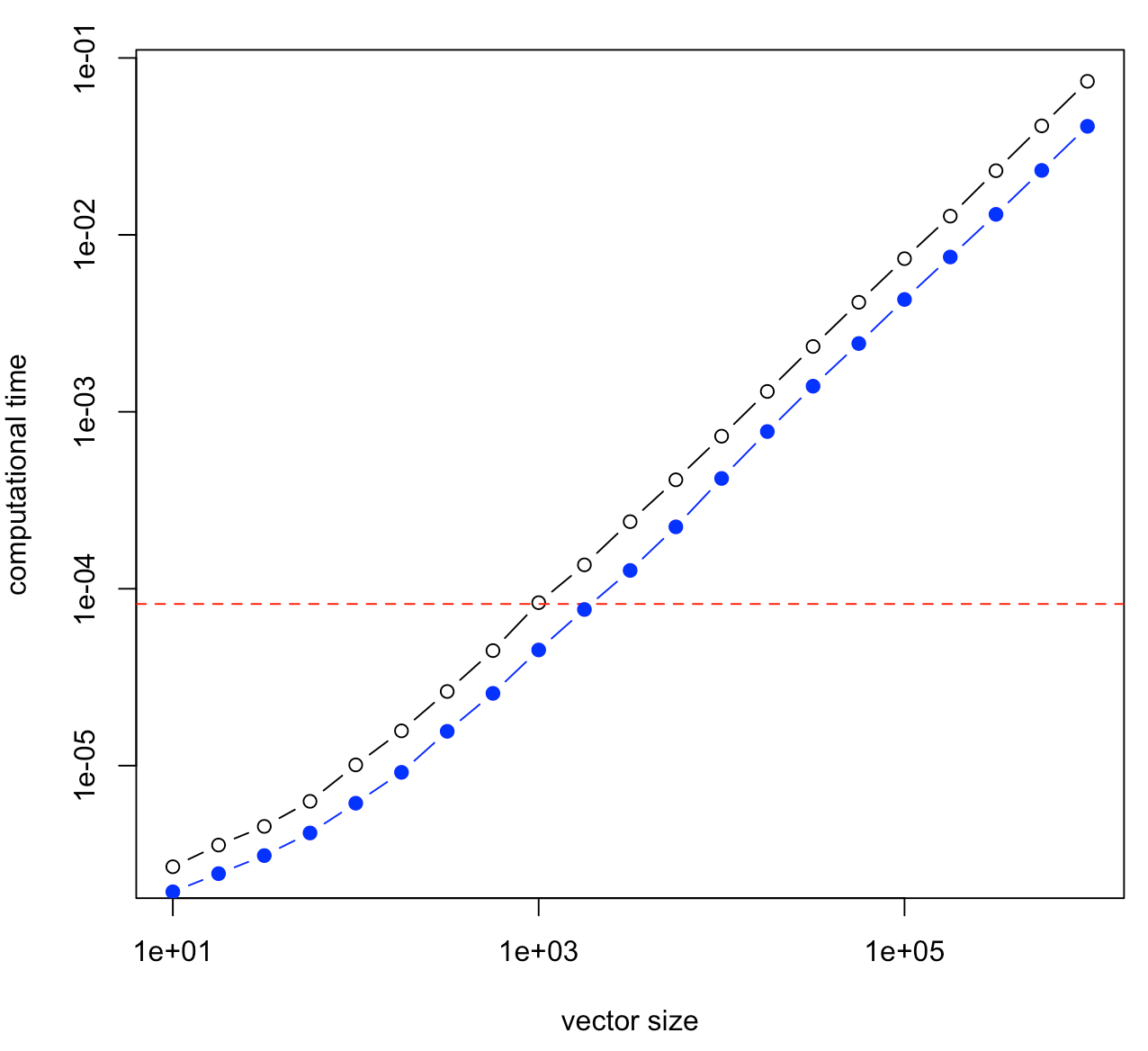Want to share your content on R-bloggers? click here if you have a blog, or here if you don't.

Tuesday, we got our conference “Insurance, Actuarial Science, Data & Models” and Dylan Possamaï gave a very interesting concluding talk. In the introduction, he came back briefly on a nice discussion we usually have in economics on the kind of model we should consider. It was about optimal control. In many applications, we start with a one period economy, then a two period economy, and pretend that we can extend it to $n$ period economy. And then, the continuous case can also be considered. A few years ago, I was working on sports game as an optimal effort startegy (within in a game – fixed time). It was with a discrete model, I was running simulations to get an efficient frontier, where coaches might say “ok, now we have enough (positive) difference, and we get closer to the end of the game, so we can ‘lower the effort’ i.e. top players can relax a little bit” (it was on basket-ball games). I asked a good friend of mine, Romuald, to help me on some technical parts of proofs, but he did not like so much my discrete-time model, and wanted to move to continuous time. And for now six years, we keep saying that someday we should get back to that paper….

My initial thoughts were that the difference was really “cultural”: you are either a continuous-time sort of guy, or a discrete-time one (or maybe none of the two, but that’s another problem). He works with stochastic processes, I work with time series. Of course, we can find connections, but most of the time, the techniques are very different. And tuesday, Dylan mentioned a very nice illustration that it’s not necessarily a cultural difference, and sometimes, it is great to move to continuous time. So I wanted to illustrate that idea.

Consider for instance the following curve.

vu = seq(0,1,length=601)
vv = sin(vu*pi)
plot(vu,vv,type="l",lwd=2)The goal is to find the value of the maximum, numerically. And here, there are two (very) different strategies

• the discrete one: we see a (finite) collection of points – for instance, the graph above is a collection of 601 points (connected with a straight line) – and in that case, we need a standard algorithm (in $O(n)$) to get the value of the maximum
• the continuous one: we see a function $x\mapsto \sin(\pi x)$, and in that case, we use optimization routines

In the second case, use for instance

optim(0,function(x) -sin(pi*x))
$par  0.5$value
 -1

For the first case, we can use the standard R function, and see how long it takes to use simulations to get an approximation of the maximum

library(microbenchmark)
max_time = function(n) median(microbenchmark(max(sin(runif(n)*pi)))$time) vn = 10^(seq(1,6,length=21)) vt = Vectorize(max_time)(vn) plot(vn,vt/1e9,col="blue",pch=19,type="b",log="xy") but of course, some home-made code can also be used c_max = function(n=100){ x = sin(runif(n)*pi) y = x for(i in 2:length(x)) { if(x[i] > y) { y = x[i] }} return(y)} max_time=function(n) median(microbenchmark(c_max(n))$time)
lines(vn,vt/1e9,type="b")We can add that horizontal red line using

abline(h=median(microbenchmark(optim(.5,function(x) sin(pi*x)))\$time)/1e9,lty=2,col="red")


So, indeed, it looks like computational time to find the maximum in a list of $n$ elements is linear in $n$, i.e. $O(n)$. And R code is faster than home-made code. But also, interestingly, using continus time (based on analysis techniques) can be much faster. So, sometimes, considering continuous time models can be much easier to solve, from a numerical perspective.